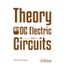Theory on DC electric circuits

• ISBN:

• Formato:

De: \$45.000,00Por: \$38.250,00ou 48X de \$796,87Economia de \$6.750,00\$('.plugin-preco').price(307333);Theory on DC electric circuits
De: \$45.000,00
Por: \$38.250,00ou
48x de \$796,87
sem juros
Vendedor Libreria de la U
307333

This book is intended as a major support for the OC Electric Circuits course from the Electrical Engineering program and the Automation Engineering program at Universidad de La Salle. Its main contribution is to provide the students with a step-by-step explanation and detailed illustrations about the main concepts and analysis techniques 0f DC electrie circuits and their related measurement systems.

Atributos LU

Título Theory on DC electric circuits Alejandro Sánchez Salcedo Introduction 1. Electric Risk 1.1. Common electric risk factors 1.2. Effects of current in human beings 1.3. Electric impedance of the human body Exercises 2. Significant figures2.1. Rules of significant figures 2.2. Mathematical operations with significant figures Exercises 3. System of Units 3.1. International System of Units 3.2. Base units 3.3. Derived units 3.4. Multiples and sub-multiples3.5. Printing rules Exercises 4. Scientific Notation 4.1. Mathematical operations with scientific notation4.2. Scientific notation and significant figures 4.3. Printing rules Exercises 5. Electrical quantities 5.1. Electric charge 5.2. Electric current  5.3. Voltage 5.4. Power 5.5. Energy Excercises 6. Electric Circuit 6.1. Circuit elements6.2. Electric circuit 6.3. Circuit concepts 6.4. Circuit analysisExercises 7. Ohm's Law 7.1. Electric resistance 7.2. Rated power Exercises 8. Kirchhoff's Laws 8.1. Kirchhoff's Current Law (KCL) 8.2. Kirchhoff's Voltage Law (KVL) 8.3. Delta-wye and wye-delta transformations Exercises 9. Methods of Analysis 9.1. Nodal analysis 9.2. Mesh analysis Exercises 10. Operational Amplifier 10.1. Ideal OP AMP 10.2. Inverting amplifier 10.3. Non-inverting amplifier 10.4. Voltage follower 10.5. Summing amplifier 10.6. Difference amplifier 10.7. Cascaded OP AMP circuits  10.8. Non-ideal OP AMP Exercises 11. Circuit Theorems11.1. Linearity theorem 11.2. Superposition principie11.3. Source transformation11.4. Thévenin's theorem 11.5. Norton's theorem 11.6. Relationship between Thévenin's and Norton's theorems 11.7. Maximum power transfer theorem Exercises 12. Capacitors and Inductors 12.1. Capacitor 12.2. Inductor Exercises 13. First-Order Circuits 13.1. Solution of first-order differential equation13.2. Forcing functions: Singularity functions 13.3. First-order circuits Exercises 14. Second Order Circuits 14.1. Solution of second-order differential equation Excercises 15. Introduction to Measurement Systems in DC Circuits 15.1. Accuracy 15.2. Precision 15.3. Uncertainty 15.4. Error 15.5. Specifications of digital measurement instruments 15.6. Measurement of DC current  15.7. Measurement of OC voltage 15.8. Measurement of resistance 15.9. Measurement of power Exercises References Libro 9789588939926 2016 234 380 16.5 x 23.5 cm Tapa Rústica